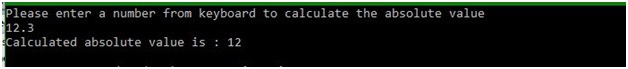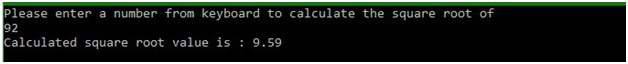# abs() and sqrt() functions of math.h in C

In this article, we are going to know about the abs() and sqrt() functions of math.h header file in C language and learn the process to use them.
Submitted by Manu Jemini, on March 17, 2018

Mathematics is something that always easy if you know the correct trick. So here we are learning the most common trick to solve some important calculations like finding the absolute value and getting the square root of a number.

To calculate the square root of a number you should use the function sqrt(), which will return a number representing the square root of the number that you have passed.

Similarly, you can use the abs() function which will return a number representing the absolute value of a number.

These functions are from the math.h file, which should be included in the program to use these functions. Below, you got two example for each one, if you want more clarification.

## math.h - abs() function Example in C

﻿

```#include <stdio.h>
#include <math.h>

int main()
{
// set the type of variable
int number, a;

// message for user
printf("Please enter a number from keyboard to calculate the absolute value\n");
scanf("%d", &number);

// storing the absolute value
a = abs(number);

// printing the calculated value
printf("Calculated absolute value is : %d\n", a);

return 0;
}
```

Output## math.h - sqrt() function Example in C

﻿

```#include <stdio.h>
//to use 'sqrt()' function
#include <math.h>

int main()
{
// set the type of variable
float number, a;

// message for user
printf("Please enter a number from keyboard to calculate the square root of\n");
scanf("%f", &number);

// storing the square root of entered number
a = sqrt(number);

// printing the calculated value
printf("Calculated square root value is : %.2lf\n", a);

return 0;
}
```

Output